# CCSDS Optical High Photon Efficiency Telemetry Waveform Generation

This example shows how to generate a high photon efficiency (HPE) waveform for the free space optical communications systems defined in Consultative Committee for Space Data Systems (CCSDS) 142.0-B-1 section 3 . HPE optical communications are required for applications in which power efficiency is the dominant consideration in link design. The example describes the functions of the channel coding and synchronization sublayer of the data link layer for transferring the transfer frames over an optical photon-starved space link. In these photon-starved links, the photon-efficiency of the link is the primary concern. This example shows how to generate a binary vector to be provided to the physical layer, given a set of CCSDS transfer frames produced by the data link protocol sublayer. The binary vector indicates the positions of the pulsed slots. The `1`s and `0`s of the resultant binary vector indicate pulsed and non-pulsed slots, respectively, in the optical transmission.

### Introduction

This figure shows the internal organization of the coding and synchronization sublayer of telemetry (TM) signaling at the transmitting end. The coding and synchronization sublayer accepts CCSDS transfer frames of constant code rate and data length from the data link protocol sublayer, performs the necessary functions, and delivers a binary vector to the physical layer to indicate the slots containing light pulses. The input is a sequence of fixed-length transfer frames generated from the CCSDS TM Space Data Link Protocol defined in CCSDS 132.0-B-2 , or the CCSDS Advanced Orbiting Systems (AOS) Protocol defined in CCSDS 732.0-B-3 , or the CCSDS Universal Space Link Protocol (USLP) defined in CCSDS 732.1-B-1 .### HPE Telemetry Signal Generation

This figure shows the functional blocks of the architecture of HPE TM signaling at the transmitting end performed by the coding and synchronization sublayer.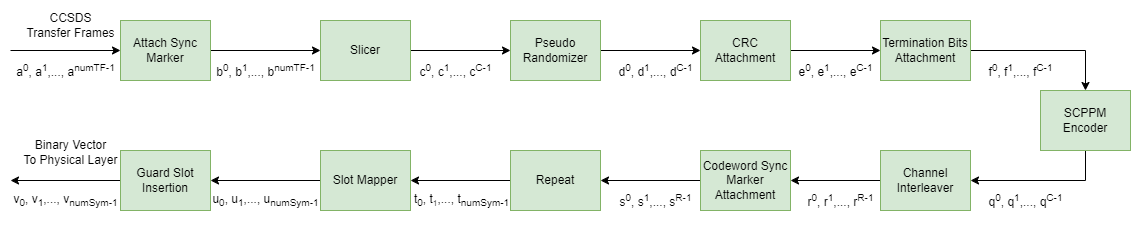The input to the coding and synchronization sublayer is a sequence of CCSDS TM, AOS, or USLP transfer frames. Consider CCSDS transfer frames ${\mathit{a}}^{0},{\mathit{a}}^{1},...,{\mathit{a}}^{\mathrm{numTF}-1}$, where `numTF` is the number of CCSDS transfer frames, and each transfer frame ${\mathit{a}}^{\mathit{i}}$ consists of 1024 bytes of data. Generate a set of 15 randomized transfer frames.

```% Generate transfer frames with random data rng("default") numTF = 15; numBytesInTF = 1024; dataTF = randi([0 1],numBytesInTF*8,numTF); % a_0,a_1,...,a_numTF-1```

Prepend a 32-bit attached synchronization marker (ASM) in hexadecimal notation, `1ACFFC1D`, to each transfer frame. A data unit that consists of an ASM and a transfer frame is a synchronization-marked transfer frame (SMTF). This figure shows the construction of SMTFs.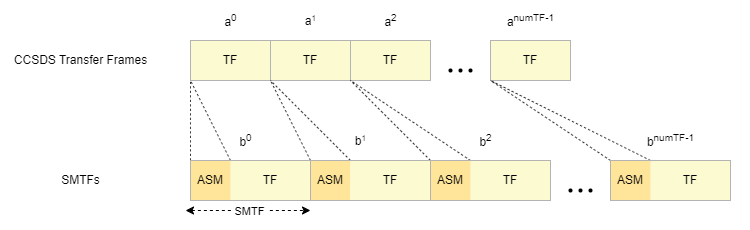Map CCSDS transfer frames to the corresponding SMTFs.

```% Attach ASM (0x1ACFFC1D) to the transfer frames ASM = [0 0 0 1 1 0 1 0 1 1 0 0 1 1 1, ... 1 1 1 1 1 1 1 0 0 0 0 0 1 1 1 0 1]'; SMTF = [repmat(ASM,1,size(dataTF,2)); dataTF]; % b_0,b_1,...,b_numTF-1```

Slice the sequence of SMTFs into information blocks of length `k`, where `k` is determined by the code rate `r`, which is a managed parameter with values from the set {1/3, 1/2, 2/3}. This table gives information on each supported code rate and its corresponding information block size. If the size of the last information block is less than `k`, then the slicer fills that block with the minimum number of `0`s (`P)` required to increase the block size to a multiple of `k`. The resultant number of information blocks after slicing is `numInfoBlocks` (C), where $\mathrm{numInfoBlocks}=\text{\hspace{0.17em}}\frac{\mathrm{numTF}+\mathit{P}}{\mathit{k}}$. This list shows the block size after slicing, in bits, for each of the supported code rates:

• Code rate 1/3 — 5006 bits

• Code rate 1/2 — 7526 bits

• Code rate 2/3 — 10046 bits

This figure shows the slicing and zero fill of SMTFs.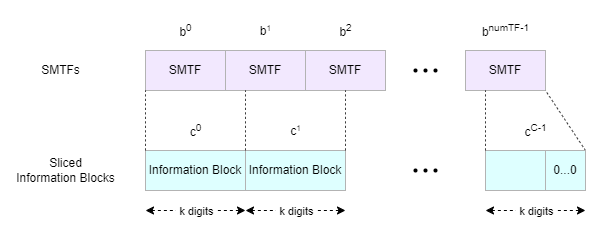Select the block size (`k`) value. The code rate (`r`) is determined based on the block size (`k`) value.

```k =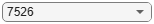7526; P = k - rem(length(SMTF(:)),k); slicerIn = [SMTF(:); zeros(P,1)]; slicerOut = reshape(slicerIn,k,[]); % c_0,c_1,...,c_C-1 numInfoBlocks = size(slicerOut,2);```

Each `k`-bit information block from the slicer is pseudo-randomized by performing the digit-wise modulo-2 addition with a pseudo-random sequence produced by a linear-feedback shift register, as specified in CCSDS 142.0-B-1 section 3.5 . The shift register is initialized to the all `1`s state at the beginning of each sliced information block.

```% Initialize pseudonoise sequence generator System object pnSequence = comm.PNSequence("SamplesPerFrame",k, ... Polynomial=[1 1 0 1 0 1 0 0 1], ... InitialConditions=ones(8,1)); % Perform pseudo randomization on SMTFs psIn = repmat(pnSequence(),1,numInfoBlocks); psOut = xor(psIn,slicerOut); % d_0,d_1,...,d_C-1```

Append 32 cyclic redundancy check (CRC) bits to the end of each pseudo-randomized information block, as described in CCSDS 142.0-B-1 section 3.6 . The CRC is used for block error detection and to terminate the serially concatenated pulse position modulation (SCPPM) decoder. Append two `0`s to each information block with attached CRC to terminate the outer convolutional encoder in the SCPPM encoder. The CRC and termination bits add a combined 34 bits to the randomized information block. This figure shows the structure of a pseudo-randomized information block with CRC and termination bits.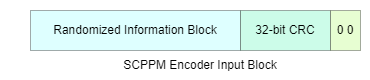Pass the resultant SCPPM encoder input block through the SCPPM encoder. The SCPPM forward error correction scheme is necessary for efficient operation of the deep space link. The encoder maps `k` + 34 bits into `S` = 15120/`m` pulse position modulation (PPM) symbols `(`where `m` is the modulation order, in the range [2, 8]).

```% Perform SCPPM encoding crc32Generator = comm.CRCGenerator(... Polynomial="x^32+x^29+x^18+x^14+x^3+1", ... InitialConditions=1); m =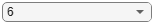6; encSym = zeros(15120/m,numInfoBlocks); for frmIdx=1:numInfoBlocks crcData = crc32Generator(psOut(:,frmIdx)); % Generate CRC scppmIn = [crcData; 0; 0]; % f_0,f_1,...,f_C-1 [encSym(:,frmIdx),info] = ccsdsSCPPMEncode(scppmIn,m); % q_0,q_1,...,q_C-1 end```

Channel interleave the sequence of PPM symbols with a convolutional interleaver, as described in CCSDS 142.0-B-1 section 3.9 . `N` and `B` are managed parameters and are so chosen that $\mathit{B}×\mathit{N}$ is a multiple of `S`, which in turn is a multiple of `N`. The interleaver has `N` rows, where an `i`th row contains a shift register of length $\mathit{i}×\mathit{B}$, consisting of $\mathit{i}×\mathit{B}$ PPM symbols. After passing the last symbol, the interleaver is operated for another $\mathit{B}×\mathit{N}×\left(\mathit{N}-1\right)$ steps before the last symbol appears at the output. Thus, the output contains $\mathit{B}×\mathit{N}×\left(\mathit{N}-1\right)$ more symbols than the input. Since $\mathit{B}×\mathit{N}×\left(\mathit{N}-1\right)$ is a multiple of `S`, there are no leftover PPM symbols in the last block. The resultant sequence `r` has $\mathit{R}\text{\hspace{0.17em}}=\text{\hspace{0.17em}}\mathit{C}+\frac{\left(\mathit{B}*\mathit{N}*\left(\mathit{N}-1\right)\right)}{\mathit{S}}$ blocks, each containing `S` symbols.

```% Channel interleaving N = 36; B = 420; channelInterleaver = comm.ConvolutionalInterleaver("NumRegisters",N, ... "RegisterLengthStep",B); % Add B*N*(N-1) zeros for the last symbol to appear at the output chInterleaveOut = channelInterleaver([encSym(:); zeros(B*N*(N-1),1)]); % r_0,r_1,...,r_R-1```

Prepend a code synchronization marker (CSM) of `W` PPM symbols based on `m` to each interleaved codeword.

``` % CSM Specification if m == 2 CSM = [0 3 1 2 1 3 2 0 0 3 2 1 0 2 1 3 1 0 3 2 3 2 1 0]; elseif m == 3 CSM = [0 3 1 2 5 4 7 6 6 7 4 5 2 1 3 0]; else CSM = [0 2 7 14 1 2 15 5 8 4 10 2 14 3 14 11]; end % Attach CSM to each S symbol block csmIn = reshape(chInterleaveOut,15120/m,[]); csmOut = [repmat(CSM',1,size(csmIn,2)); csmIn]; % s_0,s_1,...,s_R-1```

Repeat each PPM symbol `qd` times, where `qd` is a managed parameter with values from the set {1, 2, 3, 4, 8, 16, 32}. Use the repetition factor to increase the level of soft information for the decoder and to aid super-symbol synchronization. The output of the repeater has `numSym` symbols, where $\mathrm{numSym}\text{\hspace{0.17em}}=\text{\hspace{0.17em}}\mathrm{qd}×\mathit{R}×\left(\mathit{S}+\mathit{W}\right)$.

```% Repeat each PPM symbol qd =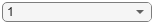1; repOut = repelem(csmOut(:),qd,1); % t_0,t_1,...,t_T-1```

Map each PPM symbol to a binary vector of length `M` (where $\mathit{M}={2}^{\mathit{m}}$) by placing (`M —` 1) `0`s and a single `1` into one of the possible positions, based on the code symbol value. M-ary PPM provides excellent power efficiency for the photon-starved operating regime of deep space optical links. After each set of `M` slots, insert $\frac{\mathit{M}}{4}$ guard slots. Guard slots are used for compatibility with existing laser technology, and to aid in synchronization. Each transmitted PPM symbol occupies $\frac{5×\mathit{M}}{4}$ PPM slots, which are transmitted over the physical channel. This figure shows slot mapping and guard slot insertion.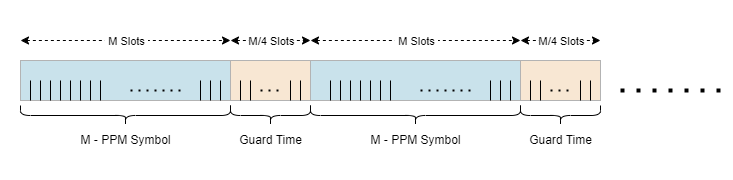After slot mapping and guard slot insertion, the result is a slot sequence ${\mathit{v}}_{0},{\mathit{v}}_{1},...,{\mathit{v}}_{\mathrm{numSym}-1}$, where each ${\mathit{v}}_{\mathit{j}}$ is a vector of length $\frac{5×\mathit{M}}{4}$.

```% Slot mapping and guard slot insertion M = 2^m; txOut = zeros(length(repOut)*5*M/4,1); mapIdx = (0:length(repOut)-1)'*5*M/4 + repOut + 1; txOut(mapIdx) = 1; % v_0,v_1,...,v_T-1```

This table shows the managed parameters for the HPE telemetry signaling.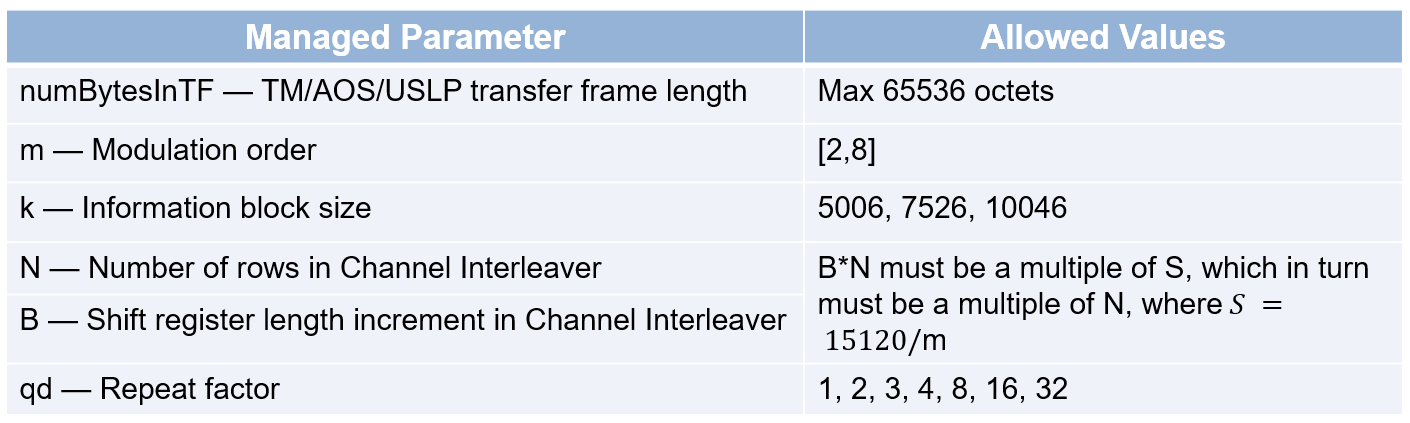### Signal Visualization

Modulate the binary vector received from the coding and synchronization sublayer using On-Off keying (OOK).

```Rb = 1e9; % Bit rate Tb = 1/Rb; % Bit duration sps = 10; % Samples per symbol fsamp = Rb*sps; % Sampling frequency unrz = ones(1,sps); % Signal for unipolar Non-return-to-zero (NRZ) sigLen = 1000; % Number of bits to visualize binData = txOut(1:sigLen); % Generate data of length sigLen modSignal = upfirdn(binData,unrz,sps); % Modulate data using OOK```

Create a `spectrumAnalyzer` object to display the frequency spectrum of the generated CCSDS optical HPE TM waveform.

```txAnalyzer = spectrumAnalyzer(SampleRate=fsamp,AveragingMethod="exponential"); txAnalyzer(modSignal)```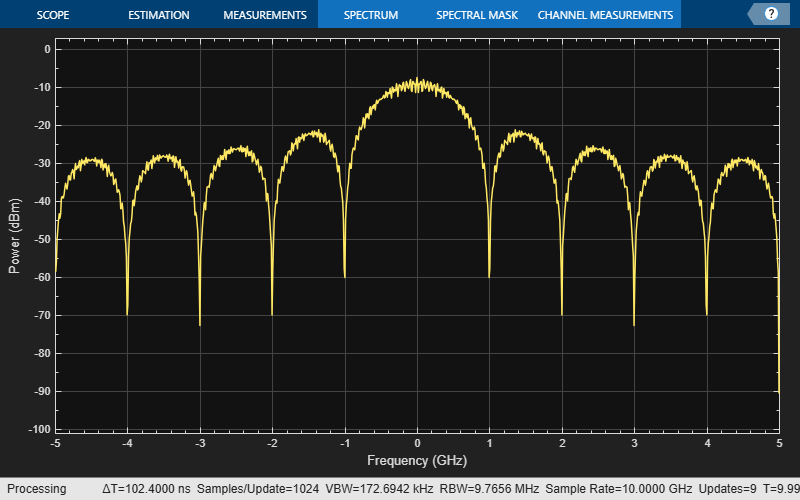Pass the modulated signal through a deep space Poisson channel.

```ns = 3; % Average signal photons per pulsed slot nb = 0.0001; % Average noise photons per slot receivedSignal = HelperDeepSpacePoissonChannel(modSignal,ns,nb);```

Create a `spectrumAnalyzer` object to display the frequency spectrum of the received CCSDS optical HPE TM waveform.

```rxAnalyzer = spectrumAnalyzer(SampleRate=fsamp,AveragingMethod="exponential"); rxAnalyzer(receivedSignal)```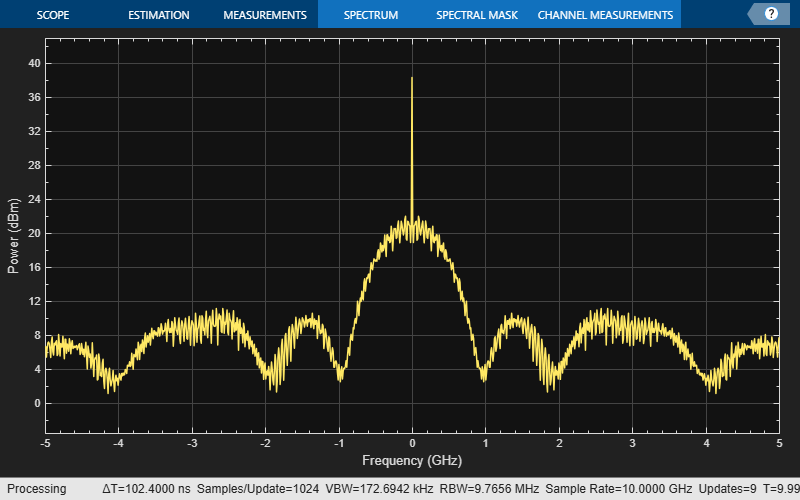### Appendix

This example uses the helper file:

`HelperDeepSpacePoissonChannel` — Add Poisson distributed noise to input signal

### References

 The Consultative Committee for Space Data Systems. Optical Communications Coding and Synchronization, Recommended Standard, Issue 1. CCSDS 142.0-B-1. Washington, D.C.: CCSDS, August 2019. https://public.ccsds.org/Pubs/142x0b1.pdf.

 The Consultative Committee for Space Data Systems. Optical Communications Physical Layer, Recommended Standard, Issue 1. CCSDS 141.0-B-1. Washington, D.C.: CCSDS, August 2019. https://public.ccsds.org/Pubs/141x0b1.pdf.

 The Consultative Committee for Space Data Systems. TM Space Data Link Protocol. Recommended Standard, Issue 2. CCSDS 132.0-B-2. Washington, D.C.: CCSDS, September 2015. https://public.ccsds.org/Pubs/132x0b3.pdf.

 The Consultative Committee for Space Data Systems. AOS Space Data Link Protocol. Recommended Standard, Issue 3. CCSDS 732.0-B-3. Washington, D.C.: CCSDS, September 2015. https://public.ccsds.org/Pubs/732x0b3e1s.pdf.

 The Consultative Committee for Space Data Systems. Unified Space Data Link Protocol. Recommended Standard, Issue 1. CCSDS 732.1-B-1. Washington, D.C.: CCSDS, October 2018. https://public.ccsds.org/Pubs/732x1b1s.pdf.